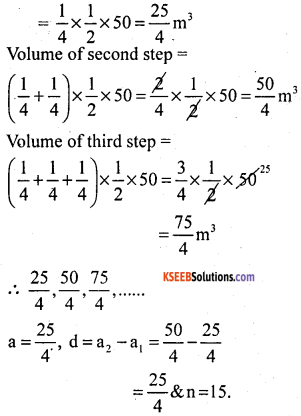# KSEEB Solutions for Class 10 Maths Chapter 1 Arithmetic Progressions Ex 1.4

Students can Download Class 10 Maths Chapter 1 Arithmetic Progressions Ex 1.4 Questions and Answers, Notes Pdf, KSEEB Solutions for Class 10 Maths helps you to revise the complete Karnataka State Board Syllabus and score more marks in your in examinations

## Karnataka State Syllabus Class 10 Maths Chapter 1 Arithmetic Progressions Ex 1.4

Question 1.
Which term of the A.P: 121, 117, 113,……, is its first negative term?
[Hint: Find n for an < 0]
The given AP is 121, 117, 113,…….
a = 121, d = 117 – 121 = – 4
Let the nth term of the AP be the first negative term.
∴ an < 0
a + ( n – 1 )d < 0
121 + (n – 1) (- 4) < 0
121 – 4n + 4 < 0
125 < 4n
4n > 125∴ n = 32.
Hence, 32nd term of the given AP is the first negative term.Question 2.
The sum of the third and the seventh terms of an AP is 6 and their product is 8. Find the sum of first sixteen terms of the AP.
Let the first term be ‘a’ and common difference is ‘d’
a3 + a7 = 6.
a + 2d + a + 6d = 6
2a + 8d = 6 divide by 2
a + 4d = 3
a = 3 – 4d → (1)
a3 × a7 = 8
(a + 2d) (a + 6d) = 8
(3 – 4d + 2d) (3 – 4d + 6d) = 8
(3 – 2d) (3 + 2d) = 8Question 3.
A ladder has rungs 25 cm apart. (see Fig.1.7 ). The rungs decrease uniformly ¡n length form 45 cm at the bottom to 25 cm at the top. If the top and the bottom rungs are 2 $$\frac{1}{2}$$ m apart, what is the length of the wood required for the rungs?
[Hint: Number of rungs $$\frac{250}{25}$$ + 1].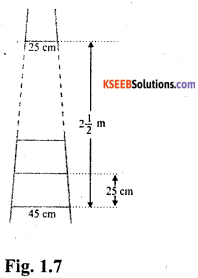Distance between top and bottom rung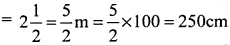Distance between every two rungs
= 25 cm [given]
Number of rungs (n) = + 1 for top most rung]
= 10 + 1 = 11
∴ n = 11
Because the rungs decrease uniformly in length from 45 cm at the bottom to 25cm at the top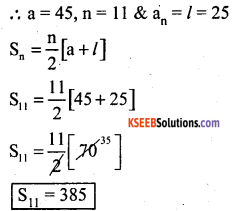Question 4.
The houses of a row are numbered consecutively from Ito 49. Show that there is a value of x such that the sum of the numbers of the houses preceding the house numbered x is equal to the sum of the numbers of the houses following it. Find this value of x.
[Hint: Sx-1 = S49 – Sx]
The numbers of houses are 1, 2, 3, 4, ……… 49
a = 1 & d = 1
sum of n terms of a AP
S = $$\frac{n}{2}$$[2a + (n – 1)d]
Sum of number of houses preceding xth house is equal to Sx-1 i.e.,Sum of numbers of houses following xth house is equal to S49 – Sx.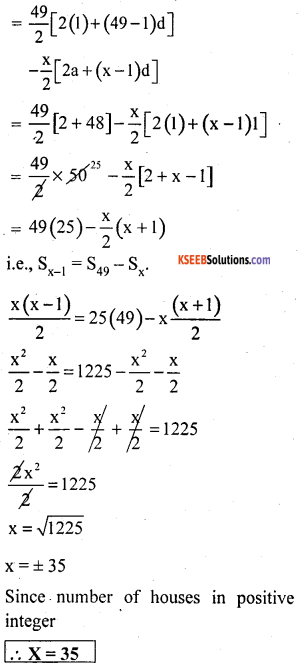Question 5.
A small terrace at a football ground comprises of 15 steps each of which is 50 m long and built of solid concrete. Each step has a rise of $$\frac{1}{4}$$ m and a tread of $$\frac{1}{2}$$ m (see Fig 5.8). Calculate the total volume of concrete required to build the terrace.
Hint: Volume of concrete required to build the first step = $$\frac{1}{4} \times \frac{1}{2} \times 50 \mathrm{m}^{3}$$Volume of concrete required to build the first step length × breadth × Height.